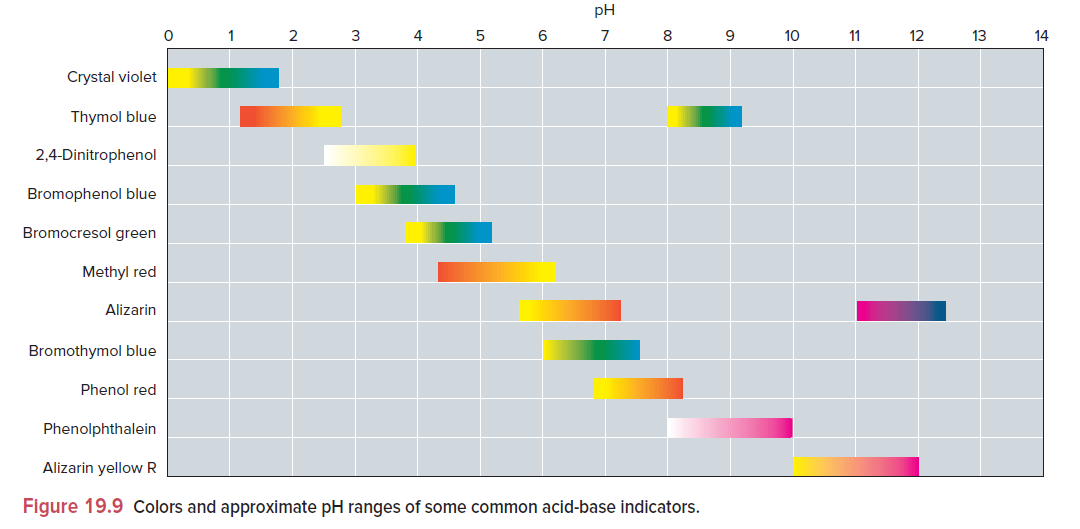# Problem: Use Figure 19.9 to find an indicator for these titrations: (a) 0.25 M C6H5COOH (see Appendix C) with 0.25 M KOH

###### FREE Expert Solution

Weak acid + Strong base titration:

• benzoic acid → C6H5COOH → Ka = 6.5 x 10-5 → weak acid
• NaOH → strong base

At equivalence point: pH > 7

• molesacid = molesbase
• final volume of base = twice initial volume
• final volume of acid = half initial volume

pKa = pH halfway from equivalence point:

${\mathbf{pK}}_{\mathbf{a}}\mathbf{=}\frac{\mathbf{1}}{\mathbf{2}}\mathbf{pH}\phantom{\rule{0ex}{0ex}}\phantom{\rule{0ex}{0ex}}\overline{){\mathbf{pH}}{\mathbf{=}}{\mathbf{2}}\mathbf{\left(}{\mathbf{pK}}_{\mathbf{a}}\mathbf{\right)}}\phantom{\rule{0ex}{0ex}}\phantom{\rule{0ex}{0ex}}\mathbf{pH}\mathbf{=}\mathbf{2}\mathbf{\left(}\mathbf{-}\mathbf{log}\left[{K}_{a}\right]\mathbf{\right)}\phantom{\rule{0ex}{0ex}}$

90% (488 ratings)###### Problem Details

Use Figure 19.9 to find an indicator for these titrations:

(a) 0.25 M C6H5COOH (see Appendix C) with 0.25 M KOH